# Texas Go Math Grade 6 Lesson 4.3 Answer Key Applying Multiplication and Division of Rational Numbers

Refer to our Texas Go Math Grade 6 Answer Key Pdf to score good marks in the exams. Test yourself by practicing the problems from Texas Go Math Grade 6 Lesson 4.3 Answer Key Applying Multiplication and Division of Rational Numbers.

## Texas Go Math Grade 6 Lesson 4.3 Answer Key Applying Multiplication and Division of Rational Numbers

Question 1.
Some friends went out to dinner. The bill was $14.50. Charles paid for $$\frac{3}{5}$$ of the entire bill. How much did Charles pay? Show how to solve the problem two different ways. Answer: The first way: We will first convert decimal to a fraction and then multiply. After that we will write the product in simplest form: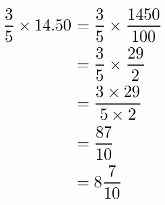Charlic paid is$ 8$$\frac{7}{10}$$
The second way:
We will convert fraction to a decimal and then multiply.
$$\frac{3}{5}$$ × 14.50 = 0.6 × 14.50 = 8.7
We can notice that Charlie paid $8.7. Question 2. Shaneeka is saving up to buy a camera that costs$65.60. She has already saved $$\frac{3}{4}$$ of the amount she needs for the camera. How much has Shaneeka saved so far? Explain your solution.
In order to calculate how much Shaneeka saved, we need to multiply 65.60 by $$\frac{3}{4}$$. We will convert the decimal to a fraction and then multiply.
After that we will write the product in simplest form.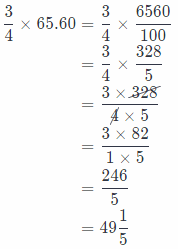So, Shaneeka saved 49$$\frac{1}{5}$$

Question 3.
Serena charges $6.50 an hour to walk dogs. She worked 5 hours last week and 9.5 hours this week. How much did she make altogether in the last two weeks? Show your work. Answer: We actually need to calculate the value of the following expression to find how much Serena made altogether in the last two weeks. (5 ÷ 9.5) ÷ 6.50 First we will add to find the total working hours in the last two weeks. 5 + 9.5 = 14.5 Now, we will multiply 14.5 by 6.50: 14.5 × 6.50 = 94.25 We can see that Serena made 94.25 in the last two weeks. Texas Go Math Grade 6 Lesson 4.3 Guided Practice Answer Key Solve. Show your work. Question 1. Chanasia has 8.75 gallons of paint. She wants to use $$\frac{2}{5}$$ of the paint to paint her living room. How many gallons of paint will Chanasia use? Answer: Convert the fraction to a decimal $$\frac{2}{5}$$ × 8.75 = 0.4 × 8.75 Multiply 0.4 × 8.75 = 3.5 Chanasia use 3.5 gallons of point Question 2. Bob and Cheryl are taking a road trip that is 188.3 miles. Bob drove $$\frac{5}{7}$$ of the total distance. How many miles did Bob drive? Answer: Convert the fraction to a decimal $$\frac{5}{7}$$ × 188.3 = 0.71 × 188.3 Multiply 0.71 × 188.3 = 133.7 Bob drives 133.7 miles Question 3. The winner of a raffle will receive $$\frac{3}{4}$$ of the money raised from raffle ticket sales. The raffle raised$530.40. How much money will the winner of the raffle get?
We need to multiply $$\frac{3}{4}$$ by 530.40 in order to find how much money the winner of ruffle will get. We will convert the decimal to a fraction and then multiply. After that we will write the product in simplest form.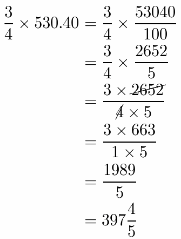So, the winner of the raffle will get $397$$\frac{4}{5}$$. Question 4. Multistep An object that weighs 1 pound on Earth weighs only 0.17 pound on the Moon. Zach’s dog weighs 17$$\frac{1}{2}$$ pounds on Earth. How much more does his dog weigh on Earth than on the Moon? Answer: We will calculate how much Zach’s dog weighs on the Moon multiplying 17$$\frac{1}{2}$$ by 0.17 We will first convert the decimal to a fraction and then multip1y After that we will write the product in the simplest form.So, Zach’s dog weights 2$$\frac{39}{40}$$ pounds on the Moon. Now we will calculate the difference between Zach’s dog weight on Earth and on the Moon.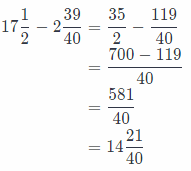Conclusion is that Zach’s dog weights for 14$$\frac{21}{40}$$ pounds on the Earth more than on the Moon. Question 5. Naomi has earned$54 mowing lawns the past two days. She worked 2.5 hours yesterday and 4.25 hours today. If Naomi is paid the same amount for every hour she works, how much does she earn per hour to mow lawns?
We actually need to calculate the value of the following expression to find how much earns per hour to mow lawns:
51 ÷ (2.5 + 4.25)
First, we will add to find the total working hours of Naomi:
2.5 + 1.25 = 6.75
Now, we will divide 51 by 6.75. but first we need to change the divisor to a whole number by multiplying by a power of 100, and then multiply the dividend by the same power of 100 and get:Conclusion is that Naomi earns $8 per hour. Question 6. Harold bought 3 pounds of red apples and 4.2 pounds of green apples from Blue Star Grocery, where both kinds of apples are$1.75 a pound. How much did Harold spend on apples?
The total weight of all apples is:
3 + 4.2 = 7.2
Multiply
7.2 × 1.75 = 12.6
Harold spent $12.6 on apples Essential Question Check-In Question 7. How can you solve a problem that involves multiplying or dividing a fraction by a decimal? Answer: The first way is that we can convert the decimal to a fraction and then multiply. The second way is that we can convert fraction to a decimal and then multiply Convert the decimal to a fraction or convert fraction to a decimal Samuel and Jason are brothers. They sell cans to a recycling center that pays$0.40 per pound of cans. The table shows the number of pounds of cans that Samuel and Jason sold for several days.Question 8.
Samuel wants to use his earnings from Monday Wednesday 12.5 and Tuesday to buy new batteries for his video game device. The batteries cost $5.60 each. How many batteries can Samuel buy? Show your work. Answer: We actually need to calculate the value of the following expression to find how many bateries Samuel can buy: (16.2 + 11.8) ÷ 5.60 First, we will add to find how much money Samuel has: 16.2 + 11.8 = 28 Now, we will divide 28 by 5.60. but first we need to change the divisor to a whole number by multiplying by a power of 100, and then multiply the dividend by the same power of 100 and get: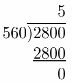Conclusion is that Samuel can buy 5 batteries. Question 9. Jason wants to use his earnings from Monday and Tuesday for online movie rentals. The movies cost$2.96 each to rent. How many movies can Jason rent? Show your work.
We actually need to calculate the value of the following expression to find how many movies Jason can rent:
(11.5 + 10.7) ÷ 2.96
First, we will add to find how much money Jason has:
11.5 + 10.7 = 22.2
Now, we will divide 22.2 by 2.96, but first we need to change the divisor to a whole number by multiplying by a power of 100. amid then multiply the dividend by the same power of 100 and get:The result is 7.5, so, conclusion is that Jason can rent 7 movies.

Question 10.
Multistep Samuel and Jason combine their earnings from Wednesday to buy their mother a gift. They spend $$\frac{3}{4}$$ of their shared earnings on the gift. How much do they spend? Use a problem solving model to determine if there is enough left over from Wednesdays earnings to buy a greeting card that costs $3.25. Explain. Answer: If Samuel and Jason combine their earnings from Wednesday, they will have the following sum of money: 12.5 + 7.1 = 19.6 Now, we will calculate how much they spent on the gift multiplying $$\frac{3}{4}$$ by 1 9.6. We will convert decimal to a fraction and multiply.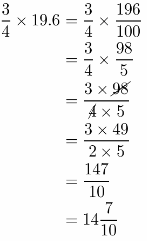So, they spent$ 14$$\frac{7}{10}$$ on the gift.
In order to determine if there is enough money to buy a greeting card, we need to subtract the sum of money which they spent on gift, 14$$\frac{7}{10}$$, from Wednesday’s earnings, which is 19.6.
19.6 – 14$$\frac{7}{10}$$ = 19.6 – 14.7 = 4.9
They have $4.9 left over from Wednesdays earnings and greeting card costs$3.25, so. they have enough money to buy greeting card.
$14$$\frac{7}{10}$$; they have enough money to buy greeting card Question 11. Eva wants to make two pieces of pottery. She needs $$\frac{3}{5}$$ pound of clay for one piece and $$\frac{7}{10}$$ pound of clay for the other piece. She has three bags of clay that weigh $$\frac{4}{5}$$ pound each. How many bags of clay will Eva need to make both pieces of pottery? How many pounds of clay will she have left over? Answer: First we will calculate how much clay she needs. We will sum $$\frac{3}{5}$$ and $$\frac{7}{10}$$ and get: $$\frac{3}{5}+\frac{7}{10}=\frac{6+7}{10}=\frac{13}{10}$$ So, she needs $$\frac{13}{10}$$ pounds of clay. Now, we will calculate how much clay she has, we will muLtiply 3 by $$\frac{4}{5}$$ and get: 3 × $$\frac{4}{5}=\frac{3 \times 4}{1 \times 5}=\frac{12}{5}$$ In order to find how many bags of clay Eva will need to make both pieces of pottery, we will divide $$\frac{13}{10}$$ by $$\frac{4}{5}$$ and get: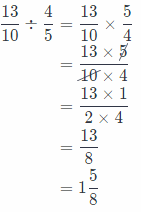So, she will need 2 bags of clay to make two pieces of pottery. Now, we will calculate how many pounds of clay she will have left over subtracting $$\frac{13}{8}$$ from $$\frac{12}{5}$$ and get: $$\frac{12}{5}-\frac{13}{8}=\frac{96-65}{40}=\frac{31}{40}$$ So, she will have $$\frac{31}{10}$$ pounds of clay left over. Question 12. Multiple Representations Give an example of a problem that could be solved using the expression 9.5 × (8 + 12.5). Answer: Samanta is paid 9.5 per hour for her work. This week she worked 12.5 hours and last week she worked 8 hours. How much money she earned in past two weeks? Tony and Alice are trying to reduce the amount of television they watch. For every hour they watch television, they have to put$2.50 into savings. The table shows how many hours of television Tony and Alice have watched in the past two months.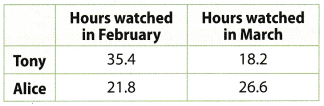Question 13.
Tony wants to use his savings at the end of March to buy video games.
The games cost $35.75 each. How many games can Tony buy? Answer: We will first calculate how much money Tony has ax. the cud of March. We will add 35.4 and 18.2 and get: 35.4 + 18.2 = 53.6 So, Tony has$ 53.6. Now, we will divide 53.6 by 35.75 to calculate how many games Tony can buy, but find we need to change the divisor to a whole number by multiplying by a power of 100. and then multiply the dividend by the same power of 100 and get: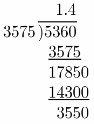So, Tony has money for one video game.

Question 14.
Alice wants to use her savings at the end of March to buy fabric for a crafts project, The fabric costs $17.50 a yard. How many full yards of fabric can Alice buy? Answer: We will first calculate how much money Alice has at the end of March. We will add 21.8 and 26.6 and get: 21.8 + 26.6 = 18.1 Alice has$ 48.4. Now, we will divide 48.4 by 17.50 to find how many full yards of fabric she can by. but first we need to change the divisor to a whole number 1w multiplying by a power of 10, and then multiply the dividend by the same power of 10:So, Alice has money to buy 2 full yards of fabric.

H.O.T. Focus On Higher Order Thinking

Question 15.
Multiple Representations You are measuring walnuts for banana walnut oatmeal and a spinach and walnut salad. You need $$\frac{3}{8}$$ cup of walnuts for the oatmeal and $$\frac{3}{4}$$ cup of walnuts for the salad. You have a $$\frac{1}{4}$$-cup scoop. Describe two different ways to find how many scoops of walnuts you will need.
First way is to add $$\frac{3}{4}$$ and $$\frac{3}{8}$$ in order to find how many cups of walnut we need.
$$\frac{3}{4}+\frac{3}{8}=\frac{6+3}{8}=\frac{9}{8}$$
Now, we will divide $$\frac{9}{8}$$ by $$\frac{1}{4}$$ and get:The other way is to convert fractions to a decimals and divide like that. Here, $$\frac{9}{8}$$ = 1.125 and $$\frac{1}{25}$$ = 0.25. but first we need to change the divisor to a whole number by multiplying by a power of 100. and then multiply the dividend by the same power of 100.  In this case we have the following: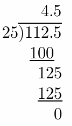So, we will need 4.5 or 4$$\frac{1}{2}$$ scoops of walnuts.

Nadia charges $7.50 an hour for babysitting. She babysits 18.5 hours the first week of the month and 20 hours the second week of the month. Question 16. Explain the Error To find her total earnings for those two weeks, Nadia writes 7.5 × 18.5 + 20 =$158.75. Explain her error. Show the correct solution.
What If? Suppose Nadia raises her rate by $0.75 an hour. How many hours would she need to work to earn the same amount of money she made in the first two weeks of the month? Explain. Answer: Nadia earned in the past two weeks following sum of money: 7.50 × (18.5 + 20) = 7.50 × 38.5 = 288.75 Now, Nadia raised her rate by$0.75 on hour. so, now she charges: